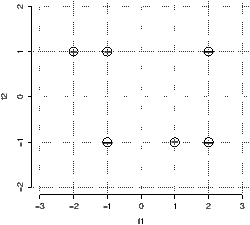# DM825 - Introduction to Machine Learning Sheet 14, Spring 2013 [pdf format]

Exercise 1

Do exercises 1, 4, 5 from Exam 2010.

Exercise 2 – Tree based methods

Consider a data set comprising 400 data points from class C1 and 400 data points from class C2. Suppose that a tree model A splits these into (300,100) assigned to the first leaf node (predicting C1 and (100,300) assigned to the second leaf node (predicting C2, where (n,m) denotes that n points come from class C1 and m points come from class C2. Similarly, suppose that a second tree model B splits them into (200,400) and (200,0), respectively. Evaluate the misclassification rates for the two trees and show that they are equal. Similarly, evaluate the pruning criterion for the cross-entropy case for the two trees.

Exercise 3 – Tree based methods

You are given the following data points: Negative: (-1, -1) (2, 1) (2, -1); Positive: (-2, 1) (-1, 1) (1,-1). The points are depicted in Figure 1.Figure 1: The data points for classification.

1. Construct a decision tree using the greedy recursive bi-partitioning algorithm based on information gain described in class. Use both criteria the Gini index and the entropy. In the search for the split threshold θ discretize the continue scale of the two features and consider only values in {−1.5,0,1.5} for f1 and {0} for f2. Represent graphically the tree constructed and draw the decision boundaries in the Figure 1. Table 1 might be useful for some computations

 x y −(x/y) · log(x/y) x y −(x/y) · log(x/y) 1 2 0.50 1 5 0.46 1 3 0.53 2 5 0.53 2 3 0.39 3 5 0.44 1 4 0.50 4 5 0.26 3 4 0.31
 Table 1: Numerical values for the computation of information gains.

2. Use the tree to predict the outcome for the new point (1,1).

Exercise 4 – Nearest Neighbor

1. Draw the decision boundaries for 1-Nearest Neighbor on the Figure 1. Make it accurate enough so that it is possible to tell whether the integer-valued coordinate points in the diagram are on the boundary or, if not, which region they are in.
2. What class does 1-NN predict for the new point: (1, 1).
3. What class does 3-NN predict for the new point: (1, 0).

Exercise 5 – Practical

Analyze by means of classification tree the data on spam email from the UCI repository. Use `rpart` from the `rpart` package and the `ctree` from the `party` package.

Exercise 6 – PCA

Using the `iris` data readily available in R use principle component analysis to identify two components and plot the data in these components. Can you classify the data at this stage?

Exercise 7 – Probability and Independence

A joint probability table for the binary variables A, B, and C is given below.

 A / B b1 b2 a1 (0.006, 0.054) (0.048, 0.432) a2 (0.014, 0.126) (0.032, 0.288)
 Table 2: Joint probability distribution P (A, B, C)

• Calculate P (B, C) and P (B).
• Are A and C independent given B? (Remember to report the justification of your answer.)